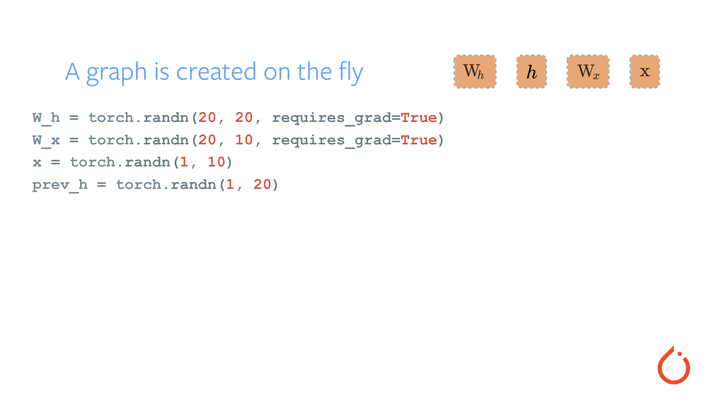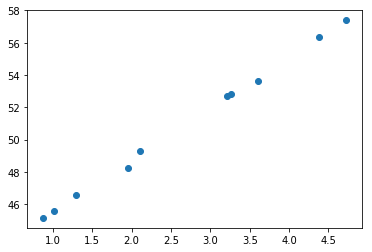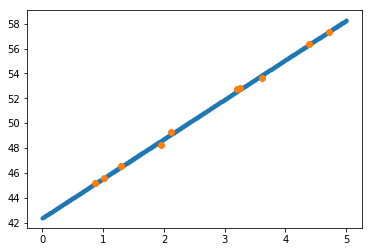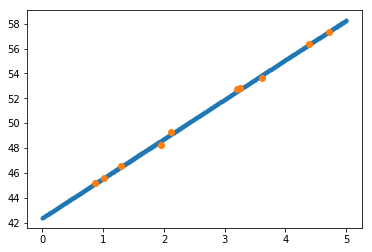# Intro to PyTorch¶

PyTorch is a deep learning package for building dynamic computation graphs.

More broadly, it's a GPU-compatible replacement for NumPy. You can think of it as NumPy + auto-differentiation.

# Basic Mechanics¶

## Tensors¶

The Tensor type is essentially a NumPy ndarray. There are several types of Tensors, each of which correspond to a NumPy dtype.

The main ones you will probably use are:

Data Type Tensor Type NumPy dtype
32-bit floating point torch.FloatTensor float32
8-bit integer (unsigned) torch.ByteTensor uint8
64-bit integer (signed) torch.LongTensor int64

In general, you want to use FloatTensor by default, unless your data is specifically an integer (in which case you'd use a LongTensor) or your data is bits (you'd want to use ByteTensor).

You can find a full list of tensor types here.

To construct a uninitialized 4x6 matrix (think malloc, so not guaranteed to be all 0), we can use:

In :
# Import PyTorch and other libraries
import torch
import numpy as np

In :
# Note that torch.Tensor is short for torch.FloatTensor
uninit_float = torch.Tensor(4, 6)
print(uninit_float)
print("Type of above Tensor (it's also printed when you print the tensor):")
print(type(uninit_float))

 2.4886e+04  4.5783e-41  2.4886e+04  4.5783e-41 -7.4654e+23  4.5782e-41
3.4307e+04  4.5783e-41  3.4312e+04  4.5783e-41 -7.4780e+23  4.5782e-41
7.2868e-44  6.1657e-44  6.8664e-44  0.0000e+00  2.4886e+04  4.5783e-41
2.4886e+04  4.5783e-41  1.5050e-38  0.0000e+00  1.5050e-38  0.0000e+00
[torch.FloatTensor of size 4x6]

Type of above Tensor (it's also printed when you print the tensor):
<class 'torch.FloatTensor'>


We can also create Tensors directly from (optionally nested) lists.

In :
some_float_tensor = torch.Tensor([3.2, 4.3, 5.5])
some_float_tensor2 = torch.Tensor(np.array([[3.2], [4.3], [5.5]]))
print(some_float_tensor)
print(some_float_tensor2)

 3.2000
4.3000
5.5000
[torch.FloatTensor of size 3]

3.2000
4.3000
5.5000
[torch.FloatTensor of size 3x1]



## Operations¶

PyTorch has a huge library of various operations (e.g. indexing, slicing, math, linear algebra, sampling, etc). They're all listed here. We'll experiment with the addition operation below.

We can add with the normal Python + operator.

In :
rand_float = torch.rand(4, 6)
other_rand_float = torch.rand(4, 6)

# Three ways to add!

# Python Operator +
print(rand_float + other_rand_float)

 0.8708  0.3786  1.4768  0.3302  0.6226  0.6731
1.2611  1.6683  0.1960  0.6373  1.0876  0.4017
1.1024  1.0168  0.1729  1.0285  1.3489  1.1807
0.8318  0.5913  1.3015  0.6518  1.2018  0.9247
[torch.FloatTensor of size 4x6]

0.8708  0.3786  1.4768  0.3302  0.6226  0.6731
1.2611  1.6683  0.1960  0.6373  1.0876  0.4017
1.1024  1.0168  0.1729  1.0285  1.3489  1.1807
0.8318  0.5913  1.3015  0.6518  1.2018  0.9247
[torch.FloatTensor of size 4x6]


Out:
 0.8708  0.3786  1.4768  0.3302  0.6226  0.6731
1.2611  1.6683  0.1960  0.6373  1.0876  0.4017
1.1024  1.0168  0.1729  1.0285  1.3489  1.1807
0.8318  0.5913  1.3015  0.6518  1.2018  0.9247
[torch.FloatTensor of size 4x6]

Broadcasting is a construct in NumPy and PyTorch that lets operations apply to tensors of different shapes. Under certain conditions, a smaller tensor can be "broadcast" across a bigger one. This is often desirable to do, since the looping happens at the C-level and is incredibly efficient in both speed and memory.

In the example below, x has shape (3,) and y has shape (5, 3). We can still add them together --- the smaller tensor is automatically added to each row of the larger tensor.

In :
# Random LongTensors from 0 to 9.
x = torch.LongTensor(3).random_(10)
y = torch.LongTensor(5, 3).random_(10)

print(x)
print(y)
print(x+y)

 4
6
0
[torch.LongTensor of size 3]

9  3  7
1  6  6
8  6  4
9  1  5
2  8  6
[torch.LongTensor of size 5x3]

13   9   7
5  12   6
12  12   4
13   7   5
6  14   6
[torch.LongTensor of size 5x3]



Broadcasting, if used improperly, can also lead to inadvertent bugs.

Consider this example: Say you want to multiply a matrix of shape (4, 6) with one of shape (6, 4) to get something of shape (4, 4). You might be tempted to use the * operator, which is for elementwise multiplication. For matrix multiplication, we use either Tensor.mm or the @ operator.

However, broadcasting leads to a particularly nasty bug that is hard to detect due to broadcast (this behavior is thankfully being deprecated by PyTorch).

In :
x = torch.LongTensor(4, 6).random_(10)  # [4,6]
y = torch.LongTensor(6, 4).random_(10)  # [6,4]

print("x: ", x)
print("y: ", y)

# Matrix multiply
print("x @ y (matrix multiply) : ", x @ y)

# USUALLY UNINTENTIONAL ELEMENTWISE-MULTIPLICATION
print("x * y (elementwise multiply) : ", x * y)

x:
8  9  3  4  0  4
2  6  2  6  3  2
3  7  2  2  5  8
7  8  7  1  6  8
[torch.LongTensor of size 4x6]

y:
0  1  1  3
1  4  8  2
5  9  2  9
4  7  0  2
8  3  7  8
3  5  8  0
[torch.LongTensor of size 6x4]

x @ y (matrix multiply) :
52  119  118   77
70  105   91   72
89  118  162   85
119  167  191  150
[torch.LongTensor of size 4x4]

x * y (elementwise multiply) :
0   9   3  12   0  16
16  12  10  54   6  18
12  49   0   4  40  24
49  64  21   5  48   0
[torch.LongTensor of size 4x6]


/home/kaiyuzh/pyenvs/py3/lib/python3.5/site-packages/torch/tensor.py:309: UserWarning: self and other not broadcastable, but have the same number of elements.  Falling back to deprecated pointwise behavior.
return self.mul(other)


A big part of programming with tensors is keeping track of the expected shapes of your tensors and whether these shapes are actually showing up --- doing so will dramatically reduce the amount of bugs you have.

## Reshaping¶

It's often desirable to reshape a Tensor, maybe to broadcast with something else or to turn it into something that is easier to reason about. We can do that with the .view function.

In :
x = torch.LongTensor(4, 4).random_(10)
print(x)

# Turn it into a Tensor of shape (2, 8)
y = x.view(2, 8)
print(y)

# Turn it into a Tensor of shape (8, ?).
# The -1 is inferred from the shape of the Tensor.
z = x.view(8, -1)
print(z)

# Turn it into a Tensor of shape (16,) (flatten it).
# This is the same as x.view(16).
flat = x.view(-1)
print(flat)

 4  7  6  4
9  3  2  5
1  0  5  6
5  9  6  0
[torch.LongTensor of size 4x4]

4     7     6     4     9     3     2     5
1     0     5     6     5     9     6     0
[torch.LongTensor of size 2x8]

4     7
6     4
9     3
2     5
1     0
5     6
5     9
6     0
[torch.LongTensor of size 8x2]

4
7
6
4
9
3
2
5
1
0
5
6
5
9
6
0
[torch.LongTensor of size 16]



## Slicing and Indexing¶

PyTorch follows the same conventions that NumPy uses for array slicing and indexing. Here's a good intro to slicing and indexing in NumPy.

In :
x = torch.LongTensor(3, 5).random_(10)
print(x)

# Get the first row
print("First row:")
print(x)

# Get the last row
print("Last row:")
print(x[-1])

# Get the 3rd column
print("3rd column from left:")
print(x[:, 2])

# Get the last column
print("Last column from left:")
print(x[:, -1])

 3  0  0  7  8
3  5  8  2  2
7  7  6  0  2
[torch.LongTensor of size 3x5]

First row:

3
0
0
7
8
[torch.LongTensor of size 5]

Last row:

7
7
6
0
2
[torch.LongTensor of size 5]

3rd column from left:

0
8
6
[torch.LongTensor of size 3]

Last column from left:

8
2
2
[torch.LongTensor of size 3]



# Computation Graphs¶

A computation graph is simply a way to define a sequence of operations to go from input to model output.

You can think of the nodes in the graph as representing operations, and the edges in the graph represent tensors going in and out.

For example, say we wanted to build a linear regression model. This has the form $\hat y = Wx + b$.

In this equation, $x$ is our input, $W$ is a learned weight matrix, $b$ is a learned bias, and $\hat y$ is the predicted output.

As a computation graph, this looks like:When implementing models, you're basically designing and specifying computation graphs. It's a bit like playing with Legos in that you're stringing together a bunch of blocks (the operations) to achieve a final desired output.

# Variables and Autograd¶

One of PyTorch's key features (and what makes it a deep learning library) is the ability to specify arbitrary computation graphs and compute gradients on them automatically.

The main abstraction it uses to do this is torch.autograd.Variable. A Variable wraps a tensor and stores:

• The data of the underlying tensor (accessed with the .data member)
• The gradient with regards to this Variable (accessed with the .grad member)
• The function that created it (accessed with the .grad_fn member)

You can think of the Variable as a container, keeping track of the 3 items above.

In :
# Variable is in the autograd module
from torch.autograd import Variable

# Variables wrap tensors
x = Variable(torch.Tensor([1, 2, 3]), requires_grad=True)
# You can access the underlying tensor with the .data attribute
print(x.data)

# Any operation you could use on Tensors, you can use on Variables
# Operations between Variables produce Variables.
y = Variable(torch.Tensor([4, 5, 6]), requires_grad=True)
z = x + y
print(z.data)

# But z also stores where it came from!

 1
2
3
[torch.FloatTensor of size 3]

5
7
9
[torch.FloatTensor of size 3]



A note on the requires_grad argument: with most NN code, you don’t want to set requires_grad=True unless you explicitly want the gradient w.r.t. to your input. In this example, however, requires_grad=True is necessary because otherwise there would be no gradients to compute, since there are no model parameters.

Let's do some more operations and calculate the gradient.

In :
z_sum = torch.sum(z)
print(z_sum)

Variable containing:
21
[torch.FloatTensor of size 1]

<torch.autograd.function.SumBackward object at 0x7f9fe724e318>


Say we want to calculate the derivative of the sum w.r.t. the first element of x (in math, $\frac{\partial z_{sum}}{\partial x_0}$).

Autograd knows that: $$z_{sum} = x_0 + y_0 + x_1 + y_1 + x_2 + y_2$$

So the derivative of $z_{sum}$ w.r.t $x_0$ is 1! Similarily, the derivative to all elements of $x$ is 1. Let's verify this with autograd.

In :
# Backprop from s backwards into the grpah
# It'll follow the chain of computation by going from grad_fn to grad_fn
# until it reaches the input.
z_sum.backward()

Variable containing:
1
1
1
[torch.FloatTensor of size 3]



Try running the block above multiple times! What do you notice happening?

The gradient in .grad accumulates each time we call .backward() --- this is convenient for some models, but we'll usually want to zero the gradient before running backpropagation when we're training our models (more on this later).

In most models we build, we'll generally want to explicitly zero-out the gradients (details forthcoming) before calling .backward()

# PyTorch models¶

At the highest level, nn.Module defines what most would refer to as a "model". It's a convenient way for encapsulating the trainable parameters of a model or a component of your model, and subclassing this class gives you Python functions for saving it, loading it etc.

When you're building your own model, you're going to subclass nn.Module. Critically, you also need to override the __init__() and forward() functions.

• In __init__(), you should take arguments that modify how the model runs (e.g. # of layers, # of hidden units, output sizes). You'll also set up most of the layers that you use in the forward pass here.
• In forward(), you define the "forward pass" of your model, or the operations needed to transform input to output. You can use any of the Tensor operations in the forward pass.

The Linear Module defines a module that is of the form $y = Ax + b$ where A and b are learnable parameters

In :
input_size = 1
output_size = 1
my_model = torch.nn.Linear(input_size, output_size)
# we can see A and b
print(list(my_model.parameters()))

[Parameter containing:
-0.8401
[torch.FloatTensor of size 1x1]
, Parameter containing:
-0.9493
[torch.FloatTensor of size 1]
]


To call the model, we treat it as a function

In :
x = Variable(torch.Tensor([,,])) # represents putting multiple data points through the model
my_model(x)

Out:
Variable containing:
-0.9493
-1.7894
-2.6294
[torch.FloatTensor of size 3x1]

# But how do we train our model?!?¶

Remember Gradient Descent and Stochastic Gradient Descent? :)

In :
import matplotlib.pyplot as plt
%matplotlib inline

# lets generate some linear data
N = 10

# params we are trying to learn
A = torch.Tensor([np.pi])
b = torch.Tensor()

x = torch.rand(N, input_size) * 5
y = A * x + b + torch.rand(N, input_size) # add some noise to spice it up

plt.scatter(x.numpy(), y.numpy())
plt.show()## But first, Loss Functions¶

Intuitively, loss functions serve to tell your model how poorly it's doing --- the purpose of training is to adjust the weights of our model to minimize the loss.

A loss function takes a true output $y$ and a model-predicted output $\hat y$ and calculates the loss. If $y = \hat y$, our model produced the correct output and thus our loss is 0. The further our predicted $\hat y$ from the true $y$, the higher our loss is.

PyTorch comes with a large collection of loss functions. A common loss used for regression problems is the mean squared error (nn.MSELoss).

In :
import torch.nn.functional as F

x_var = Variable(x)
y_var = Variable(y)

learning_rate = 0.05

num_epochs = 500
for idx in range(num_epochs):
y_hat = my_model(x_var)
loss = F.mse_loss(y_hat, y_var) # this is the mean squared error

loss.backward() # it computes the gradient for us!

for param in my_model.parameters():

if idx % 50 == 0:
print(idx, loss.data)

0 2938.597900390625
50 46.934242248535156
100 7.510673522949219
150 1.220460057258606
200 0.21682476997375488
250 0.05669074133038521
300 0.031140219420194626
350 0.02706342563033104
400 0.026413029059767723
450 0.0263094250112772

In :
xs = Variable(torch.arange(0, 5, 0.01).view(-1, 1))
ys = my_model(xs)
plt.scatter(xs.data.numpy(), ys.data.numpy(), marker='.')
plt.scatter(x.numpy(), y.numpy())
plt.show()
print(list(my_model.parameters()))[Parameter containing:
3.1788
[torch.FloatTensor of size 1x1]
, Parameter containing:
42.3802
[torch.FloatTensor of size 1]
]


# But wait, there's more!¶

## Optimizers¶

Now that we can calculate the loss and backpropagate through our model (with .backward()), we can update the weights and try to reduce the loss!

PyTorch includes a variety of optimizers that do exactly this, from the standard SGD to more advancedtechniques like Adam and RMSProp.

At construction, PyTorch parameters take the parameters to optimize. When we run an input through our model, calculate the loss, and backpropagate, the gradients are automatically stored in the parameters (since they're all Variables). With these gradients, the optimizer can update the weights.

Optimizers live in the torch.optim module.

In :
import torch.optim as optim

learning_rate = 0.05

my_model = torch.nn.Linear(input_size, output_size) # reset model
opt = optim.SGD(my_model.parameters(), lr=learning_rate)

num_epochs = 500
for idx in range(num_epochs):
y_hat = my_model(x_var)
loss = F.mse_loss(y_hat, y_var) # this is the mean squared error

loss.backward() # it computes the gradient for us!

opt.step() # this does the parameter for us!

if idx % 50 == 0:
print(idx, loss.data)

0 2490.942138671875
50 47.29664611816406
100 7.5684943199157715
150 1.2296714782714844
200 0.2182965725660324
250 0.05692403391003609
300 0.03117733635008335
350 0.02706919051706791
400 0.02641412243247032
450 0.026309436187148094

In :
xs = Variable(torch.arange(0, 5, 0.01).view(-1, 1))
ys = my_model(xs)
plt.scatter(xs.data.numpy(), ys.data.numpy(), marker='.')
plt.scatter(x.numpy(), y.numpy())
plt.show()
print(list(my_model.parameters()))[Parameter containing:
3.1788
[torch.FloatTensor of size 1x1]
, Parameter containing:
42.3802
[torch.FloatTensor of size 1]
]


As we can see, it worked!

Speical thanks to Nelson Liu for the base materials